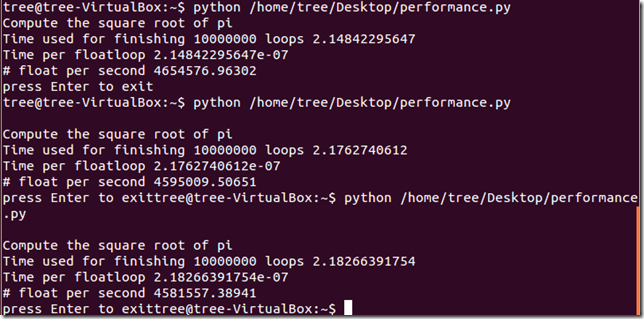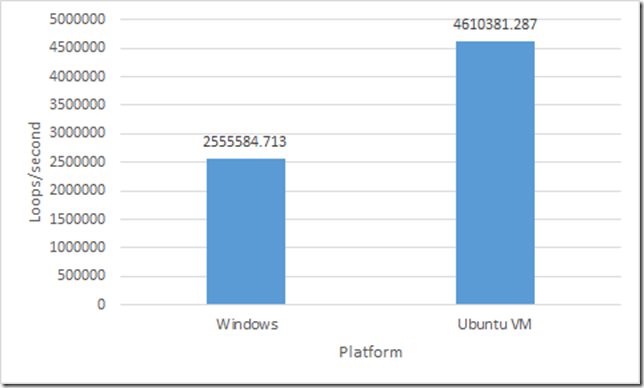1. from __future__ import division
2. import time
3. import math
4. loops = 10000000
5. begin_time = time.time()
6.
7. for i in range(loops):
8.     a = math.sqrt(math.pi)
9.     i += 1
10.
11. finish_time = time.time()
12.
13. time_used = finish_time – begin_time
14.
15. print ‘Compute the square root of pi’
16. print ‘Time used for finishing’, loops,’loops’, time_used
17. print ‘Time per floatloop’, time_used / loops
18. print# float per second’, 1/(time_used / loops)
19.
20. dummy = raw_input(‘press Enter to exit’)